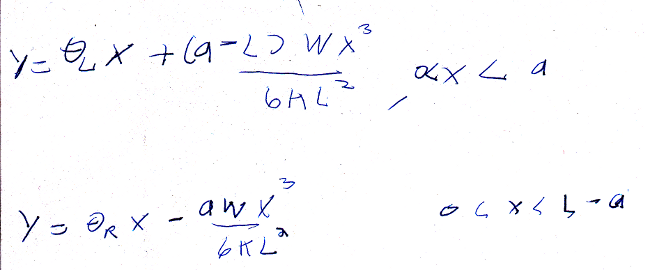# Beam Bending 6

Another worked example.

A beam supported on both sides, but not rigidly, with a weight that may be played asymmetrically.

So it’s similar to the last problem, but more complicated / general.

Last time we relied on having special insight into the problem; this time we’ll do it more generally.

I’m setting up the coordinates for this problem a little unusually.The beam runs from x = 0 to x = L.  The weight is at x = a.  The “left” half of the beam I’m treating normally, between x = 0 and x = a.

However, I’m flipping the right half around, so instead of being between x = a and x = L, it’s between x = 0 and x = L-a.

I don’t know if this seems more confusing, but my intention was to be less confusing.

The idea is that this allows me to define the angles theta-left and theta-right that are the angles the ends are positioned at,and then from there continue with the other thetas in increasing order (increasing until they meet in the middle).

I’m treating theta-L and theta-R separately from the others because they represent orientation, not bending, so they have no elastic potential energy associated with them.

This is the function F we need to find an extreme for:This looks monstrous, but we can break it down, and it becomes not so bad once we start taking gradients.

The first term is the potential energy.  Note that the gravitational term only involves half of the angles.  This is because you know the height by looking at how the beam bends to the weight on one half alone.

The first lambda-1 lagrange multiplier represents that the height at the weight should be the same whether you come from the left side or the right.

The second lambda-2 lagrange multiplier represents that the slope at the weight should be the same whether you come from the left or right.  (A zero-width kink in the beam would have infinite energy so would obviously not be energetically favorable).  Due to the way I flipped the right side, this adds a minus sign to the slope when comparing them, which is why the heights subtract to zero but the slopes add to zero.

Like I said, that expression looks scary, but it simplifies fast.  Taking the gradients with respect to theta-L and theta-R giveswhich are a simple pair of linear equations for lambda-1 and lambda-2:The gradient for angles on the left sides gives:Note that for t=1, theta=0, and (because a is less than L) increasing t decreases theta.  This is because the beam starts with bend theta-L, and as you go to the other end it eventually has to bend back up.

Similarly for thetas on the right side:We don’t know theta-L or -R yet, but it turns out those only contribute to the initial slopes.  So we can add together all the other thetas as before to get the general shape of the curve:Note the obvious symmetry in form between these two branches.  You can get the lower equation by substituting a = L – a in the upper equation.  This is because the equations have no way of “knowing” whether the weight is closer to the left side or right.

Anyway, we can use those equations themselves to solve for theta-L and -R, by matching heights and slopes (making one negative).Although it is less obvious, these thetas have the same symmetry (when replacing a with L-a) as the other equations.

So, basically, the problem is solved.  It may not be obvious that the solution is the same as Wikipedia’s, but it is.Trivia: Although the answer for this problem was a simple algebraic expression, in general determining if two different expressions are equal is undecidable.